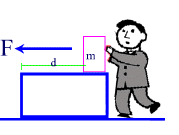#### Work and Energy

Energy is defined as :
• The ability to do work
• The amount of work done
If you push an object through a certain distance (d), using a force (F), you can calculate the amount of work done , (W) required in moving the object.
The drawing below illustrates the above mentionned variables:WORK = Force X Distance.  Note that the distance is in the same direction as the applied force.
W = F X d

Where F is the Force doing the "pushing" and d is the distance the object moves.

The distance and the force must be in the same direction.

In this equation, Force is measured in [N], Newtons and distance is measured in [m], meters.

The Work-Energy Equivalence states that the amount of work done is equivalent to the energy used tp achieve this work, and viceversa.

WORK = Energy
Measured in Joules
1 Joule = 1 Newton-meter
1 J = 1Nm

Example 1:

A shopper pushes a grocery cart with a force of 100 N continuously for 50 m.
How much energy is she using up, and how much work is she doing on the cart?

Solution:

Given: F = 100 N
d =  50 m

Find: W

W = F x d
= 100 N x 50 m = 5000 J

Therefore, the shopper uses up 5000 N of Energy in pushing the grocery cart.

At the same time, the work she is doing on the cart is also 5000N since Work = Energy.